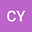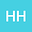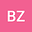Existence results for nonlinear two-parametric quantum difference equation with first-order (p,q)-derivative
•••• Changlong Yu,
• Huode Han,
• Boya Zhang
Changlong Yu
Hebei University of Science and Technology

Corresponding Author:[email protected]

Author ProfileHuode Han
Hebei University of Science and Technology
Author ProfileBoya Zhang
Hebei University of Science and Technology
Author Profile## Abstract

In this paper, we study the solvability of a nonlinear two-parametric quantum difference equation Dirichlet boundary value problem. At first, we provide and prove the formula of changing the order of integration for (p,q)-double integral. Second, We obtain the existence and uniqueness criteria of solutions for this kind of boundary value problem by using Banach contraction mapping principle, Leray-Schauder nonlinear alternative theorem and Leray-Schauder continuation theorem. At last, we give two examples to illustrate our results.